# Plane trigonometry

trigonometry in the Euclidean plane.

The elements of a triangle, its sidesand its angles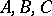(opposite to, etc.), are related in various ways. In the Euclidean plane the most important relations are the angle sum formula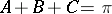(angles in radians), and the triangle inequalitiesThese inequalities are necessary and sufficient for three segments of positive length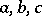to form the sides of a triangle.

Another relation is the cosine theorem: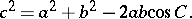In particular, when, the triangle is right-angled, and the cosine theorem becomes Pythagoras' theorem (cf. Pythagoras theorem)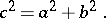In such a right-angled triangle,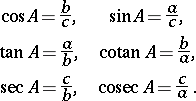In a general triangle, further relations are provided by the sine theorem: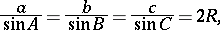whereis the radius of the circumcircle of the triangle (cf. Inscribed and circumscribed figures). A corollary of the sine theorem is the tangent formula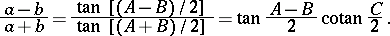With the notation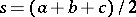for the semi-perimeter of the triangle, the following half-angle formulas follow from the cosine theorem: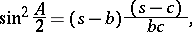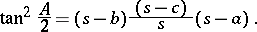## Geometry of the triangle.

Among the many remarkable lines, points and circles connected with any triangle are the circumcircle with centre 0 and radius, the incircle and the three excircles with centres,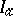,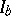,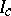and radii,,,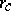(cf. Inscribed and circumscribed figures), the medians (cf. Median (of a triangle))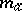,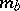,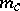, with the centroidas their common point, the inner bisectors,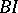,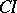, and the outer bisectors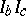,,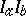, the altitude lines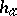,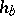,with the orthocentreas their common point, the Euler line (cf. Euler straight line) through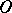,and, and the nine-point circle through the midpoints of the sides, the feet of the altitude lines, and the midpoints of the segments connecting the vertices of the triangle to its orthocentre. The nine-point circle has radius, its centreis on the Euler line betweenandsuch that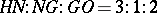, and the nine-point circle touches the incircle and the three excircles (Feuerbach's theorem).

With the notation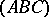for the area of the triangle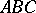, the following relations are valid: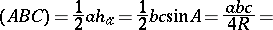It follows that, among others,Very remarkable is Morley's theorem: The points of intersection of the adjacent trisectors of the angles of any triangle form the vertices of an equilateral triangle. Indeed, a direct calculation shows that the sides of Morley's triangle have length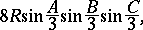which is symmetric in,and.

## The theorems of Ceva and Menelaus.

Let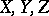be points on the (possibly extended) sidesof a triangle. Then, by the Ceva theorem,,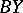and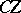are congruent if and only if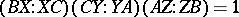(signed distances) and by the Menelaus theorem,,and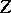are collinear if and only if.

Ptolemy's theorem (cf. Ptolemeus theorem): For any pointin the plane of a trianglethe inequality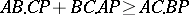holds, with equality if and only ifis on the arcof the circumcircle of(in this last case,is a circle quadrangle).

Brahmagupta's formula states that for any convex cyclic quadranglewith area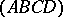, sides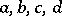and semi-perimeter, the relationholds. In general, for any quadrangle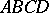, the areasatisfies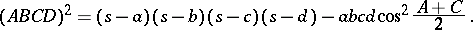It follows that among all quadrangles with given side lengths the inscribed quadrangles have maximum area (the cyclic order of the sides is immaterial).

## Regular-gons.

A regular-gon inscribed in a circle with radiushas perimeter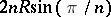and area; a regular-gon circumscribed about a circle with radiushas perimeter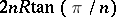and area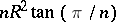. See also Regular polyhedra.